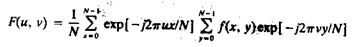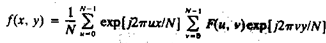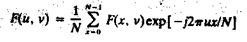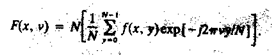13.State and prove separability property of 2D-DFT.

13.State and prove separability property of 2D-DFT.

The separability property of 2D-DFT states that, the discrete Fourier transform pair can
be expressed in the separable forms. i.e. ,(1)

For u, v = 0, 1, 2 . . . , N – 1, and(2)

For x, y = 0, 1, 2 . . . , N – 1

The principal advantage of the separability property is that F(u,v) or f(x,y) can be
obtained in two steps by successive applications of the 1-D Fourier transform or its inverse. This
advantage becomes evident if equation (1) is expressed in the form(3)

Where,(4)

For each value of x, the expression inside the brackets in eq(4) is a 1-D transform, with
frequency values v = 0, 1, . . . , N-1. Therefore the 2-D function f(x, v) is obtained by taking a
transform along each row of f(x, y) and multiplying the result by N. The desired result, F(u, v), is
then obtained by taking a transform along each column of F(x, v), as indicated by eq(3)